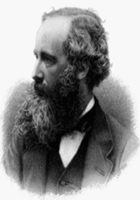# James Clerk Maxwell

(13 June 1831 – 5 November 1879 / Edinburgh, Scotland)Do you like this poet?James Clerk Maxwell was a Scottish theoretical physicist and mathematician. His most important achievement was classical electromagnetic theory, synthesizing all previously unrelated observations, experiments and equations of electricity, magnetism and even optics into a consistent theory. His set of equations—Maxwell's equations—demonstrated that electricity, magnetism and even light are all manifestations of the same phenomenon: the electromagnetic field. From that moment on, all other classic laws or equations of these disciplines became simplified cases of Maxwell's equations. Maxwell's work in electromagnetism has been called the "second great unification in physics", after ... more »

## Quotations

more quotations »

There is no comment submitted by members..
Best Poem of James Clerk Maxwell

### A Problem In Dynamics

An inextensible heavy chain
Lies on a smooth horizontal plane,
An impulsive force is applied at A,
Required the initial motion of K.

Let ds be the infinitesimal link,
Of which for the present we’ve only to think;
Let T be the tension, and T + dT
The same for the end that is nearest to B.
Let a be put, by a common convention,
For the angle at M ’twixt OX and the tension;
Let Vt and Vn be ds’s velocities,
Of which Vt along and Vn across it is;
Then Vn/Vt the tangent will equal,
Of the angle of starting worked out in the sequel.

In working the ...

Read the full of A Problem In Dynamics